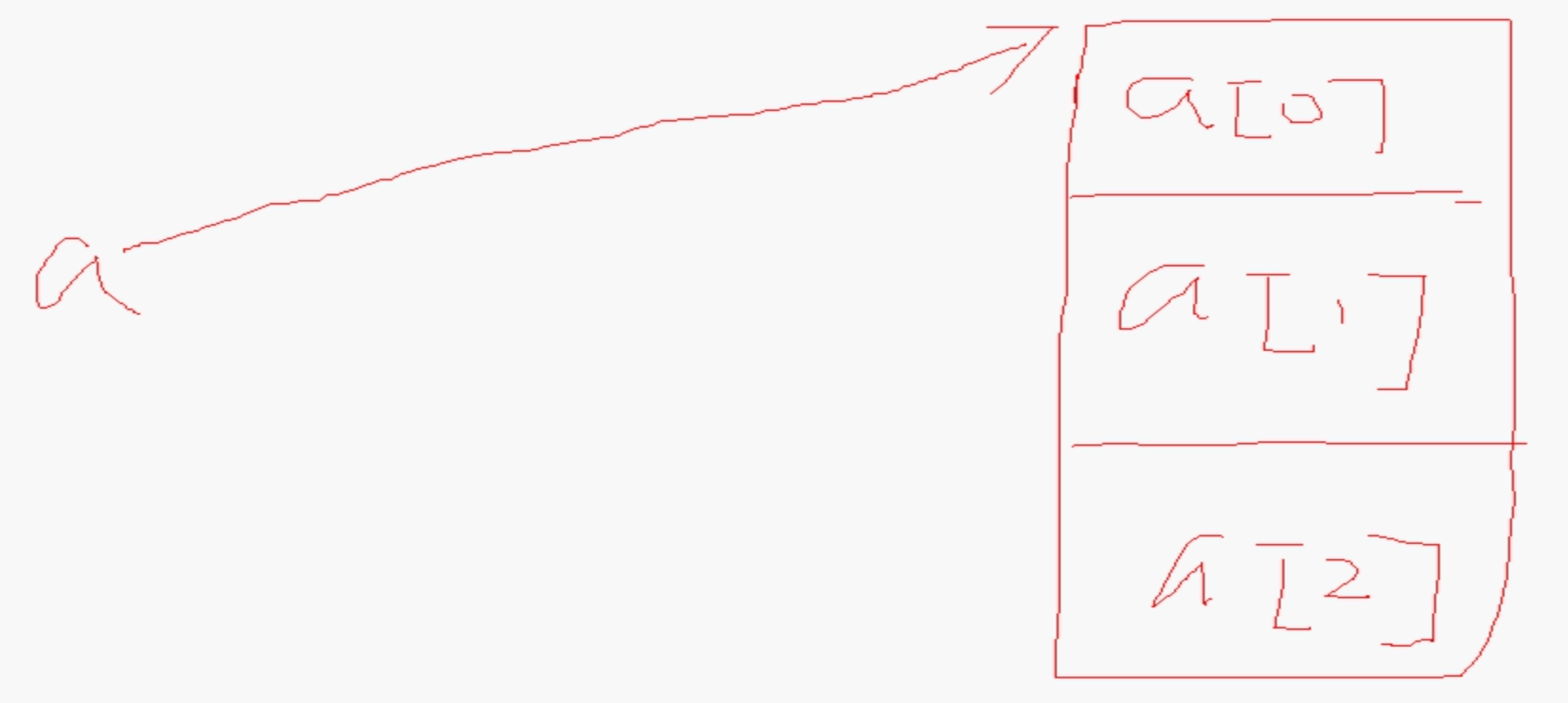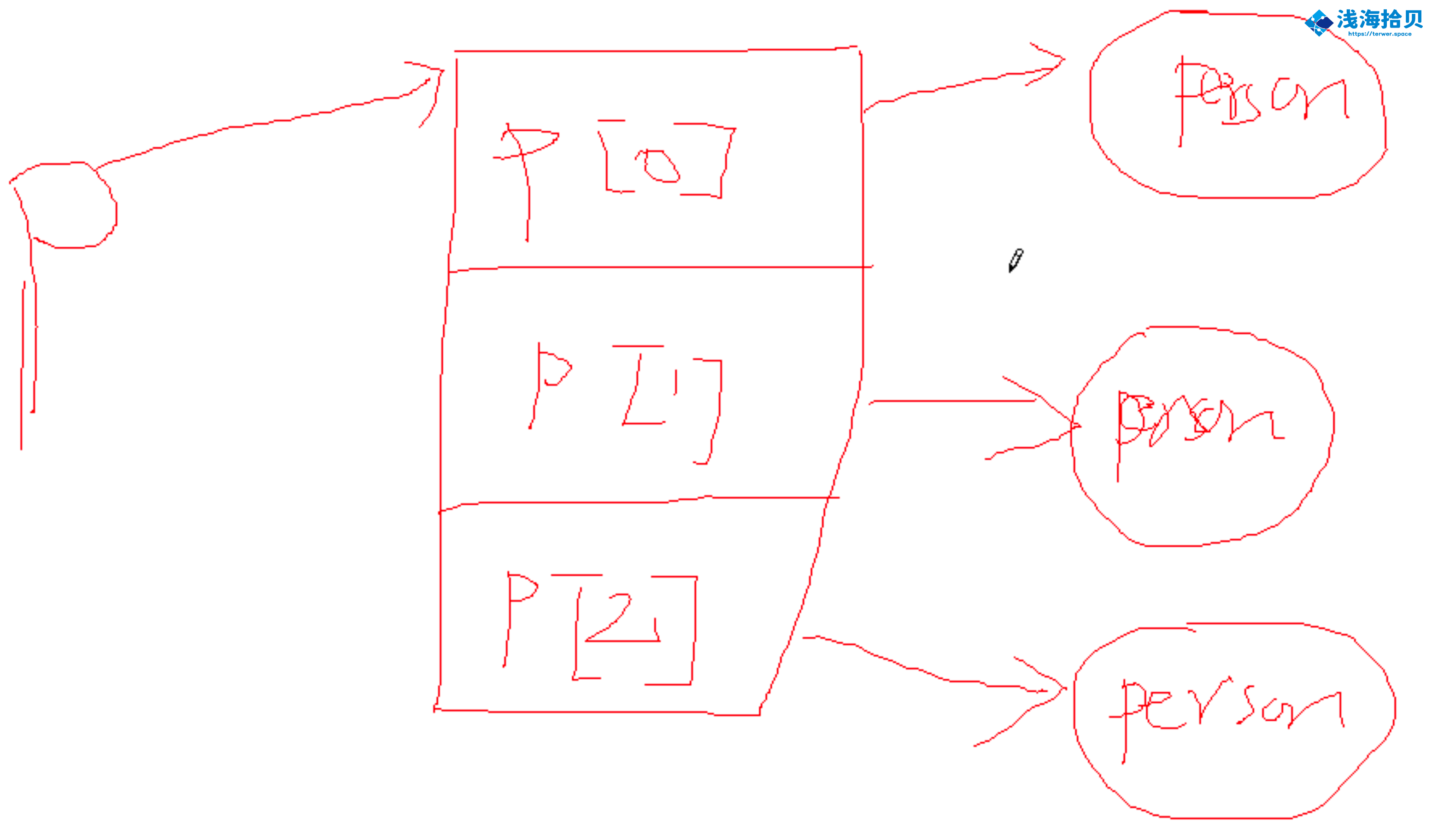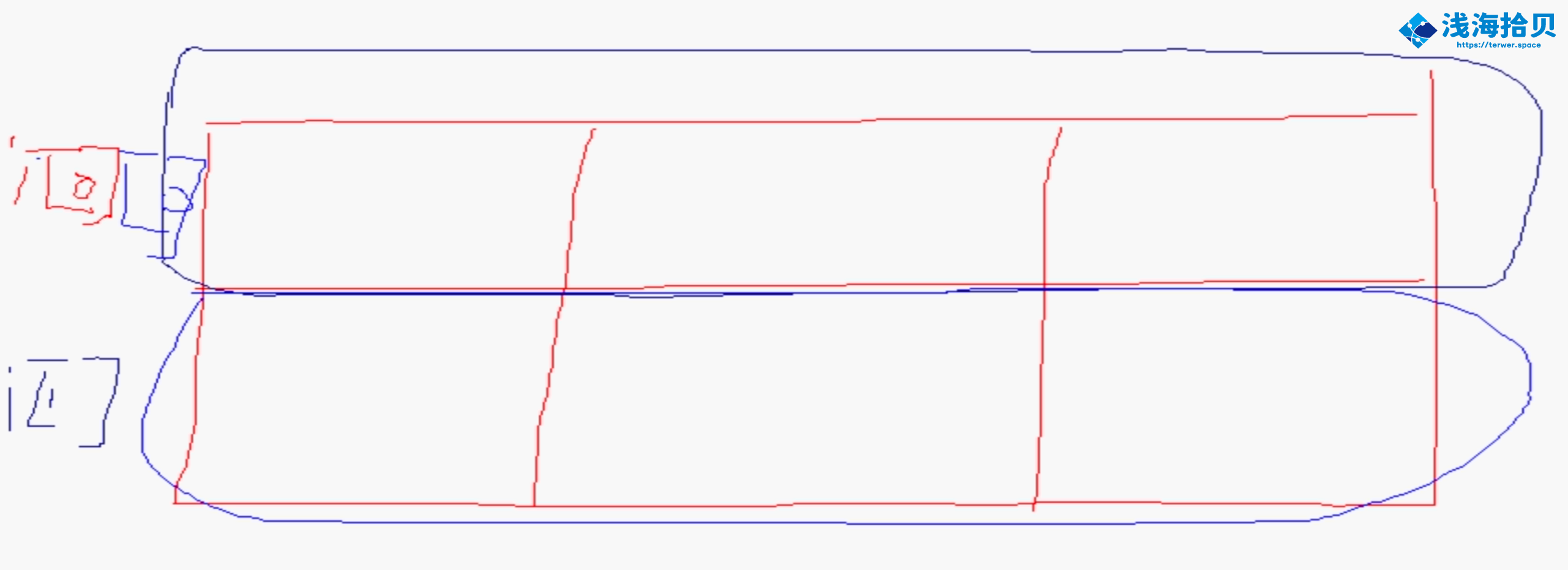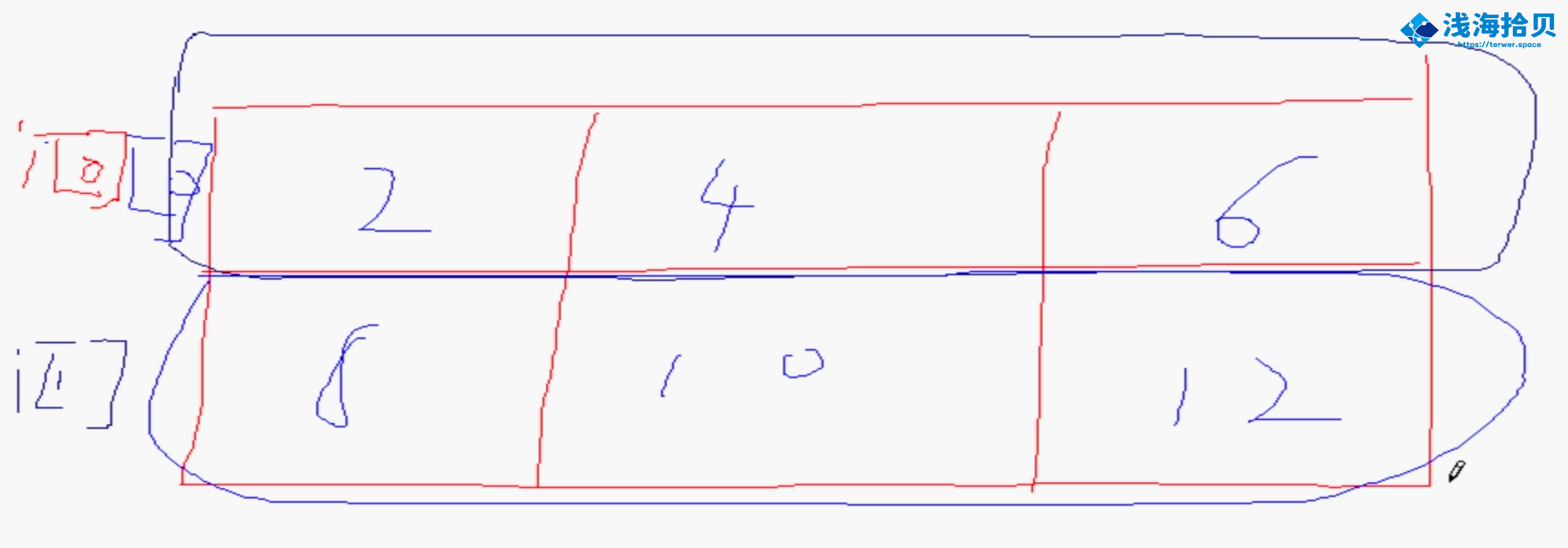# 包装类与数组

...大约 3 分钟后端开发JavaSE数组包装二维使用定义classarray

1. 包装类（Wrapper Class）。

针对原生数据类型的包装。 包装类（8 个）都位于 `java.lang`​ 包下。

java 中的 8 个包装类分别是：Byte，Short，Integer，Long，Float，Double，Character，Boolean。

他们的使用方式都是一样的，可以实现原生数据类型和包装类额双向转换。

2. 数组（Array）：相同类型 数据的集合叫做数组。

3. 如何定义数组：

``````type[] 变量名 = new type[数组中元素的个数 ];
``````

按照下列方式定义长度为 10 的数组：

``````int[] a = new int; // 或者
int a[] = new int;
``````
4. 数组中的元素索引是从 0 开始的。对于数组来说，最大的索引 == 数组的长度 - 1 。

5. 定义数组的第三种方式：

``````type[] 变量名 = new type[]{逗号分隔的初始化值列表}
``````
6. Java 中的每个数组都有一个 length 属性，他表示数组的长度。

length 属性是 public，final，int 的。数组长度一旦确定，就不能改变大小。

1. 两个数组的比较不能使用 `==`​ ，也不能使用 `equals`​ ，要使用 `Arrays.equals`​ 。

``````int[] a = {1, 2, 3};
int[] b = {1, 2, 3};
System.out.println(a==b);
System.out.println(a.equals(b));
System.out.println(Arrays.equals(a, b));

// false
// false
// true
``````
2. `int a[] = new int`​ ，其中 a 是一个引用。它指向了上次的数组对象的首地址，数组中每个元素都是 int 类型，其中仅放数据值本身。3. 下列代码的内存布局

``````Person[] p = new Person;

p = new Person(10);
p = new Person(20);
p = new Person(30);
``````4. 二维数组。二维数组是一种平面的二维结构，本质上是数组的数组。

例如下列数组，实际的存储

``````int[][] i = new int;

System.out.println(i instanceof Object);
System.out.println(i instanceof Object);
System.out.println(i instanceof int[]);

// true
// true
// true
``````5. 二维数组中初始化为偶数

``````int idx = 0;
for (int k = 0; k < 2; k++) {
for (int j = 0; j < 3; j++) {
i[k][j] = 2 * (idx + 1);
idx++;
}
}

for (int[] ints : i) {
for (int anInt : ints) {
System.out.println(anInt);
}
}
``````

如图：6. 二维数组的定义和初始化

``````/*
int[][] a = new int[];
a = new int;
a = new int;
a = new int;
*/

// int[][] a = new int[];

int[][] a = new int[][]{{1, 2, 3}, {4, 5}};

for (int[] ints : a) {
for (int anInt : ints) {
System.out.print(anInt + " ");
}
System.out.println();
}

// 1 2 3
// 4 5
``````
7. Arrays

Arrays.equals

8. System.arrayCopy

9. 三维数组。type[][][] a = new type

• 按正序
• 按倒序
• 按热度